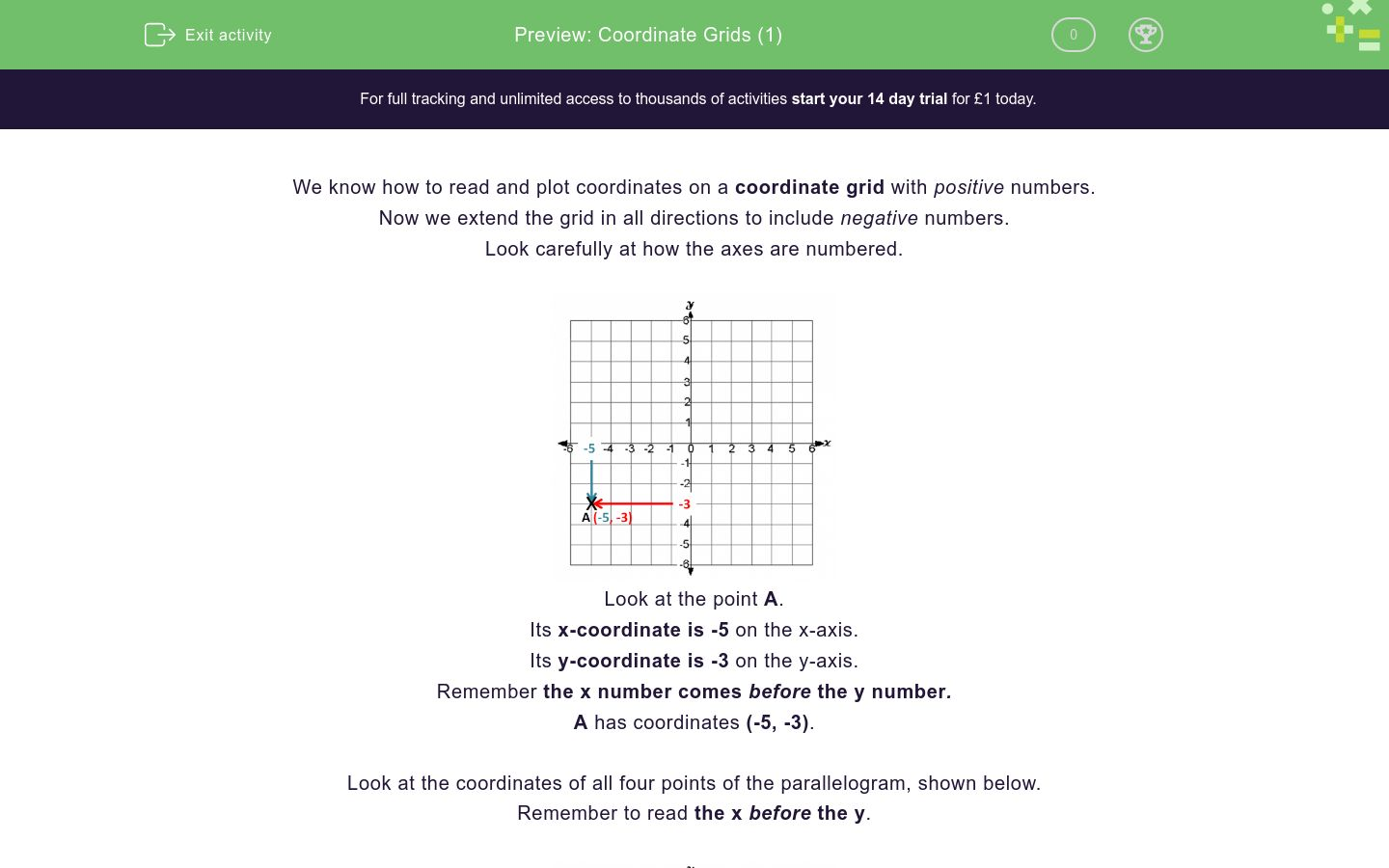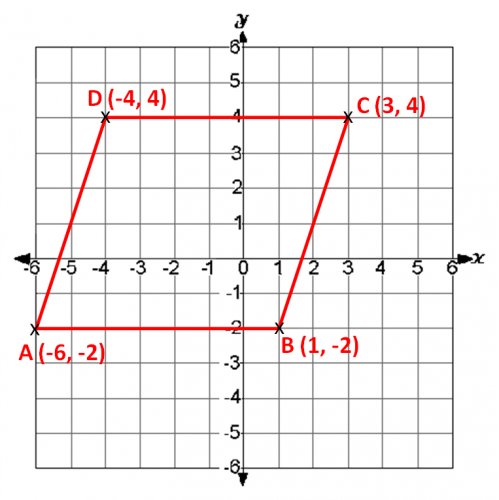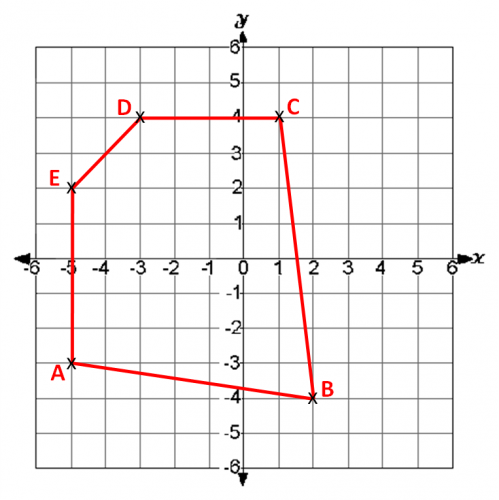# Coordinate Grids (1)

In this worksheet, students must read the coordinates of points in all four quadrants.Key stage:  KS 2

Curriculum topic:   Geometry: Position and Direction

Curriculum subtopic:   Use Coordinate Grids

Difficulty level:### QUESTION 1 of 10

We know how to read and plot coordinates on a coordinate grid with positive numbers.

Now we extend the grid in all directions to include negative numbers.

Look carefully at how the axes are numbered.Look at the point A.

Its x-coordinate is -5 on the x-axis.

Its y-coordinate is -3 on the y-axis.

Remember the x number comes before the y number.

A has coordinates (-5, -3).

Look at the coordinates of all four points of the parallelogram, shown below.

Remember to read the x before the y.Look at the coordinate grid below.

What are the coordinates of the point A?(-5, -3)

(5, -3)

(-3, -5)

Look at the coordinate grid below.

What are the coordinates of the point B?(-4, -2)

(2, -4)

(-4, 2)

Look at the coordinate grid below.

What are the coordinates of the point C?(-4, 1)

(1, 4)

(4, 1)

Look at the coordinate grid below.

What are the coordinates of the point D?(-4, 3)

(3, 4)

(-3, 4)

Look at the coordinate grid below.

What are the coordinates of the point E?(2, -5)

(-5, 2)

(-5, 4)

Look at the coordinate grid below.

What are the coordinates of the point A?(-4, -5)

(-5, 2)

(-5, -4)

Look at the coordinate grid below.

What are the coordinates of the point B?(0, -5)

(-5, 0)

(-5, -5)

Look at the coordinate grid below.

What are the coordinates of the point C?(0, -5)

(-5, 5)

(5, -5)

Look at the coordinate grid below.

What are the coordinates of the point D?(3, 4)

(4, 3)

(4, 4)

Look at the coordinate grid below.

What are the coordinates of the point E?(3, 5)

(5, -3)

(-3, 5)

• Question 1

Look at the coordinate grid below.

What are the coordinates of the point A?(-5, -3)
EDDIE SAYS
-5 on the x-axis.
-3 on the y-axis.
• Question 2

Look at the coordinate grid below.

What are the coordinates of the point B?(2, -4)
EDDIE SAYS
2 on the x-axis.
-4 on the y-axis.
• Question 3

Look at the coordinate grid below.

What are the coordinates of the point C?(1, 4)
EDDIE SAYS
1 on the x-axis.
4 on the y-axis.
• Question 4

Look at the coordinate grid below.

What are the coordinates of the point D?(-3, 4)
EDDIE SAYS
-3 on the x-axis.
4 on the y-axis.
• Question 5

Look at the coordinate grid below.

What are the coordinates of the point E?(-5, 2)
EDDIE SAYS
-5 on the x-axis.
2 on the y-axis.
• Question 6

Look at the coordinate grid below.

What are the coordinates of the point A?(-5, -4)
EDDIE SAYS
-5 on the x-axis.
-4 on the y-axis.
• Question 7

Look at the coordinate grid below.

What are the coordinates of the point B?(0, -5)
EDDIE SAYS
0 on the x-axis.
-5 on the y-axis.
• Question 8

Look at the coordinate grid below.

What are the coordinates of the point C?(5, -5)
EDDIE SAYS
5 on the x-axis.
-5 on the y-axis.
• Question 9

Look at the coordinate grid below.

What are the coordinates of the point D?(4, 3)
EDDIE SAYS
4 on the x-axis.
3 on the y-axis.
• Question 10

Look at the coordinate grid below.

What are the coordinates of the point E?(-3, 5)
EDDIE SAYS
-3 on the x-axis.
5 on the y-axis.
---- OR ----

Sign up for a £1 trial so you can track and measure your child's progress on this activity.

### What is EdPlace?

We're your National Curriculum aligned online education content provider helping each child succeed in English, maths and science from year 1 to GCSE. With an EdPlace account you’ll be able to track and measure progress, helping each child achieve their best. We build confidence and attainment by personalising each child’s learning at a level that suits them.

Get started